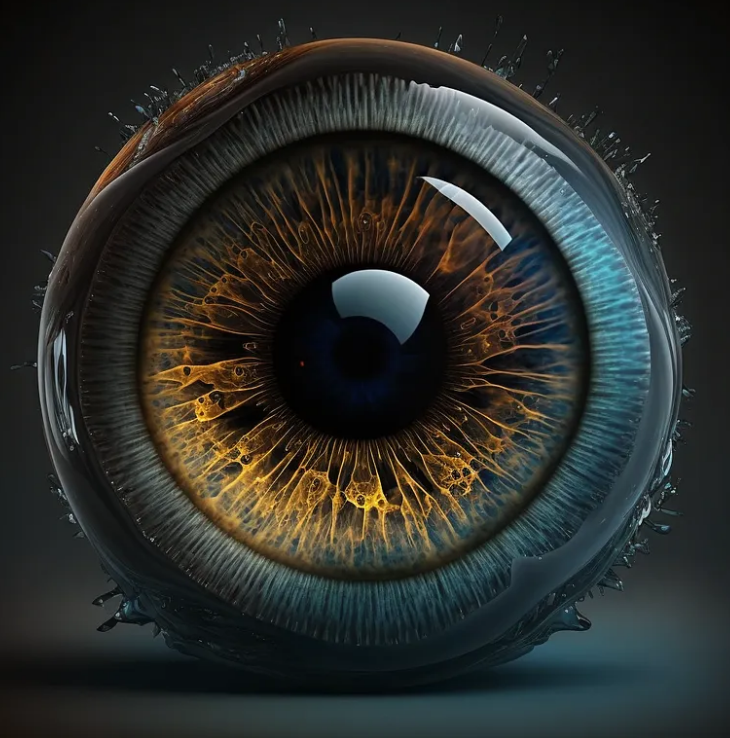Join thousands of AI enthusiasts and experts at the Learn AI Community.

## Publication# Computer Vision Tutorial Series M2C2

Last Updated on May 22, 2023 by Editorial Team

Originally published on Towards AI.

## Chapter 2— Filters

Learning Objectives:

1. What are Filters?
2. What is sobel filter?
3. How to create and apply sobel filter?
4. What is the Laplacian filter?
5. How to create and apply the Laplacian filter?

Pre-Requisites: Previous Tutorials

## What is a filter?

In computer vision, a filter is a mathematical operation that is applied to an image to transform it in some way. Filters are used to extract information from images, enhance features, remove noise, and prepare images for further processing.

There are many different types of filters used in computer vision, each with a specific purpose. Some common filters include:

1. Gaussian filter: smooths an image by removing high-frequency noise.
2. Sobel filter: detects edges in an image by computing the gradient of the image in the horizontal and vertical directions.
3. Laplacian filter: detects changes in intensity in an image by computing the second derivative of the image.
4. Median filter: removes noise from an image by replacing each pixel with the median value of its neighboring pixels.
5. Bilateral filter: smooths an image while preserving edges

Filters work by convolving an image with a small matrix, known as a kernel or a filter. The filter is centered at each pixel of the image, and the values of the neighboring pixels are multiplied by the corresponding values in the filter matrix. The resulting products are then summed, and the sum is used to replace the value of the pixel being processed.

1. Imports
`import numpy as npimport matplotlib.pyplot as plt%matplotlib inlineimport cv2`

2. Load the images in the format you want

Since we use RGB colorscheme, I will load images directly into RGB channels

`image3 = cv2.cvtColor(cv2.imread('image_path'),cv2.COLOR_BGR2RGB)`

3. Plot the image

`plt.imshow(image3)plt.set_title('Image 3')plt.show()`

for the sample I have taken the image displayed is as below

Writing a helper function to convert our image to grayScale

`def to_gray(image): return cv2.cvtColor(image,cv2.COLOR_RGB2GRAY)`

## Sobel Filter — Used to detect verticle edges

• format -> [-k,0,k]
• detect abrupt intensity changes in a certain direction(horizontal here)

4. Sobel Filter

below is the sample format of how a sobel filter looks like, if you want to learn the maths behind these filters, you can refer to this article

`sobel_x = np.array([[-1,0,1], [-5,0,5], [-1,0,1]])filtered_x = cv2.filter2D(to_gray(image3),-1,sobel_x) #represents that 'do not change the format of output image and keep it same as input image'plt.imshow(filtered_x,cmap='gray')`

output:

Let's see how the change in threshold value changes the output

`# Set a threshold, so that points above threshold will be white/visibleretval, binary_image1 = cv2.threshold(filtered_x,50,255,cv2.THRESH_BINARY)retval, binary_image2 = cv2.threshold(filtered_x,100,255,cv2.THRESH_BINARY)retval, binary_image3 = cv2.threshold(filtered_x,150,255,cv2.THRESH_BINARY)retval, binary_image4 = cv2.threshold(filtered_x,200,255,cv2.THRESH_BINARY)fig,(ax1,ax2,ax3,ax4) = plt.subplots(1,4,figsize=(15,8))ax1.set_title('threshold 50')ax1.imshow(binary_image1,cmap='gray')ax2.set_title('threshold 100')ax2.imshow(binary_image2,cmap='gray')ax3.set_title('threshold 150')ax3.imshow(binary_image3,cmap='gray')ax4.set_title('threshold 200')ax4.imshow(binary_image4,cmap='gray')`

## Why Set a Threshold?

• The purpose of using a threshold here is to remove the noise present in an image; for example, in image 1 with a threshold of 50, we detected a lot of lines, but as we increased the threshold, fewer lines were detected.
• We need a high threshold when we need only strong lines from an image and not noise.
• consider the image we are experimenting rn. Suppose that

## Sobel Filter — Used to detect horizontal edges

`format -> [-k, 0, k]`
• detect abrupt intensity changes in a certain direction(vertical here)
`sobel_y = np.array([[-1,-5,-1], [0,0,0], [1,5,1]])filtered_y = cv2.filter2D(to_gray(image3),-1,sobel_y)plt.imshow(filtered_y,cmap='gray')`
`# Set a threshold, so that points above threshold will be white/visibleretval, binary_image1 = cv2.threshold(filtered_y,50,255,cv2.THRESH_BINARY)retval, binary_image2 = cv2.threshold(filtered_y,100,255,cv2.THRESH_BINARY)retval, binary_image3 = cv2.threshold(filtered_y,150,255,cv2.THRESH_BINARY)retval, binary_image4 = cv2.threshold(filtered_y,200,255,cv2.THRESH_BINARY)fig,(ax1,ax2,ax3,ax4) = plt.subplots(1,4,figsize=(15,8))ax1.set_title('threshold 50')ax1.imshow(binary_image1,cmap='gray')ax2.set_title('threshold 100')ax2.imshow(binary_image2,cmap='gray')ax3.set_title('threshold 150')ax3.imshow(binary_image3,cmap='gray')ax4.set_title('threshold 200')ax4.imshow(binary_image4,cmap='gray')`

## Laplacian Filter

• A Laplacian filter is used to enhance edges in an image. It applies a second-order derivative kernel to an image to detect changes in intensity.
`kernel_size = 3def lap_filter(k): laplacian_filter = np.array([[1, 1, 1], [1, -k, 1], [1, 1, 1]]) laplacian_filter = laplacian_filter / np.sum(np.abs(laplacian_filter)) return laplacian_filter`
`# Apply Laplacian filterimg = cv2.imread('img_path', cv2.IMREAD_GRAYSCALE)filtered_3 = cv2.filter2D(img, -1, lap_filter(3))filtered_5 = cv2.filter2D(img, -1, lap_filter(5))filtered_7 = cv2.filter2D(img, -1, lap_filter(7))fig,(ax1,ax2,ax3) = plt.subplots(1,3,figsize=(15,8))ax1.set_title('k = 3')ax1.imshow(filtered_3,cmap='gray')ax2.set_title('k = 5')ax2.imshow(filtered_5,cmap='gray')ax3.set_title('k = 7')ax3.imshow(filtered_7,cmap='gray')`

## Wrap Up

With this, we have completed our learning objectives for this lesson.

After this, You will create filters and apply them to images of your choice.

## Upcoming

This is it for Filters and their types, next tutorial will be dedicated to Gaussian Filter

With this, we have come to the end of this article. See you at the next one

Until then, Follow for more, and don’t forget to connect with me on LinkedIn.❤❤❤

Join thousands of data leaders on the AI newsletter. Join over 80,000 subscribers and keep up to date with the latest developments in AI. From research to projects and ideas. If you are building an AI startup, an AI-related product, or a service, we invite you to consider becoming a sponsor.

Published via Towards AI